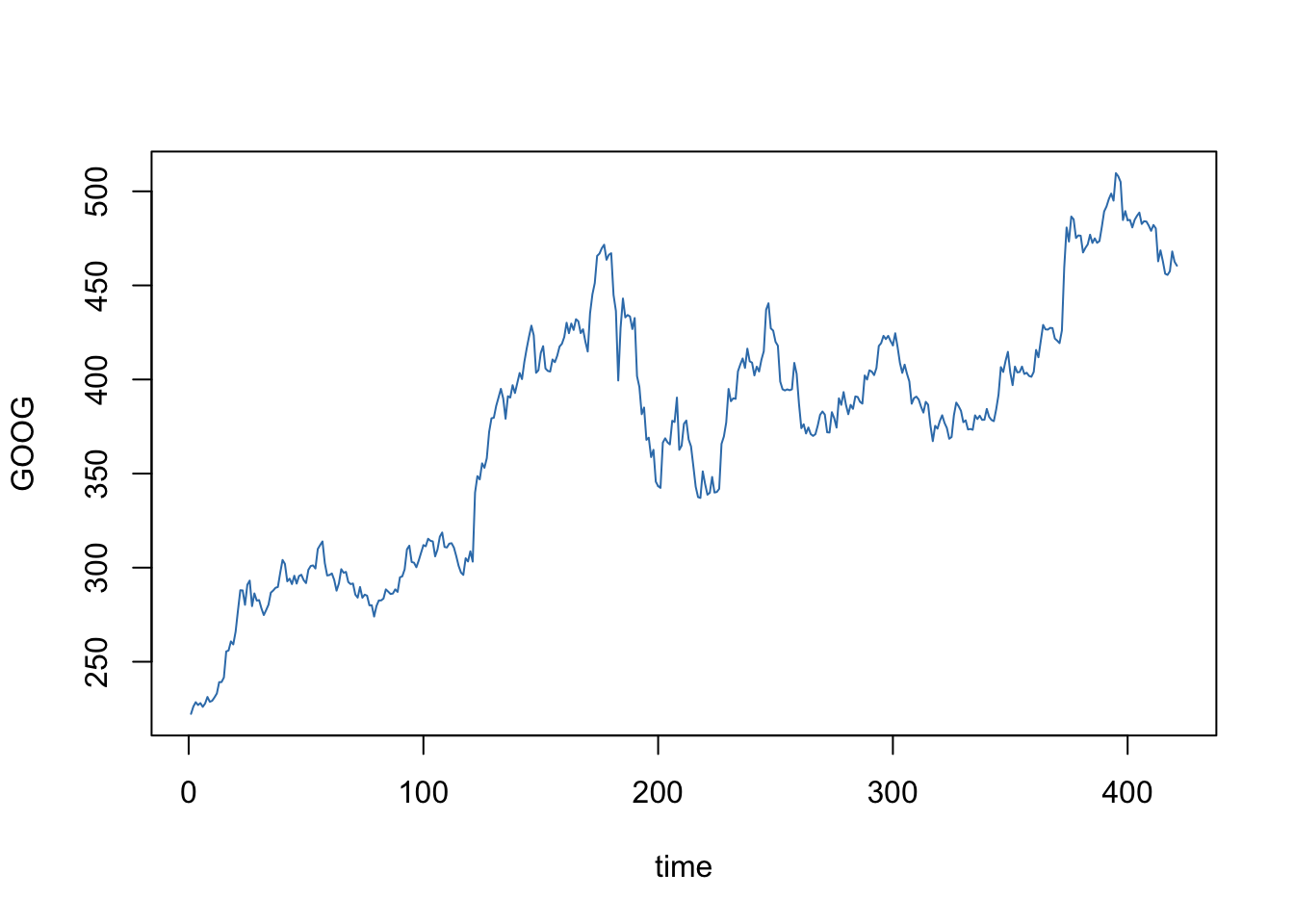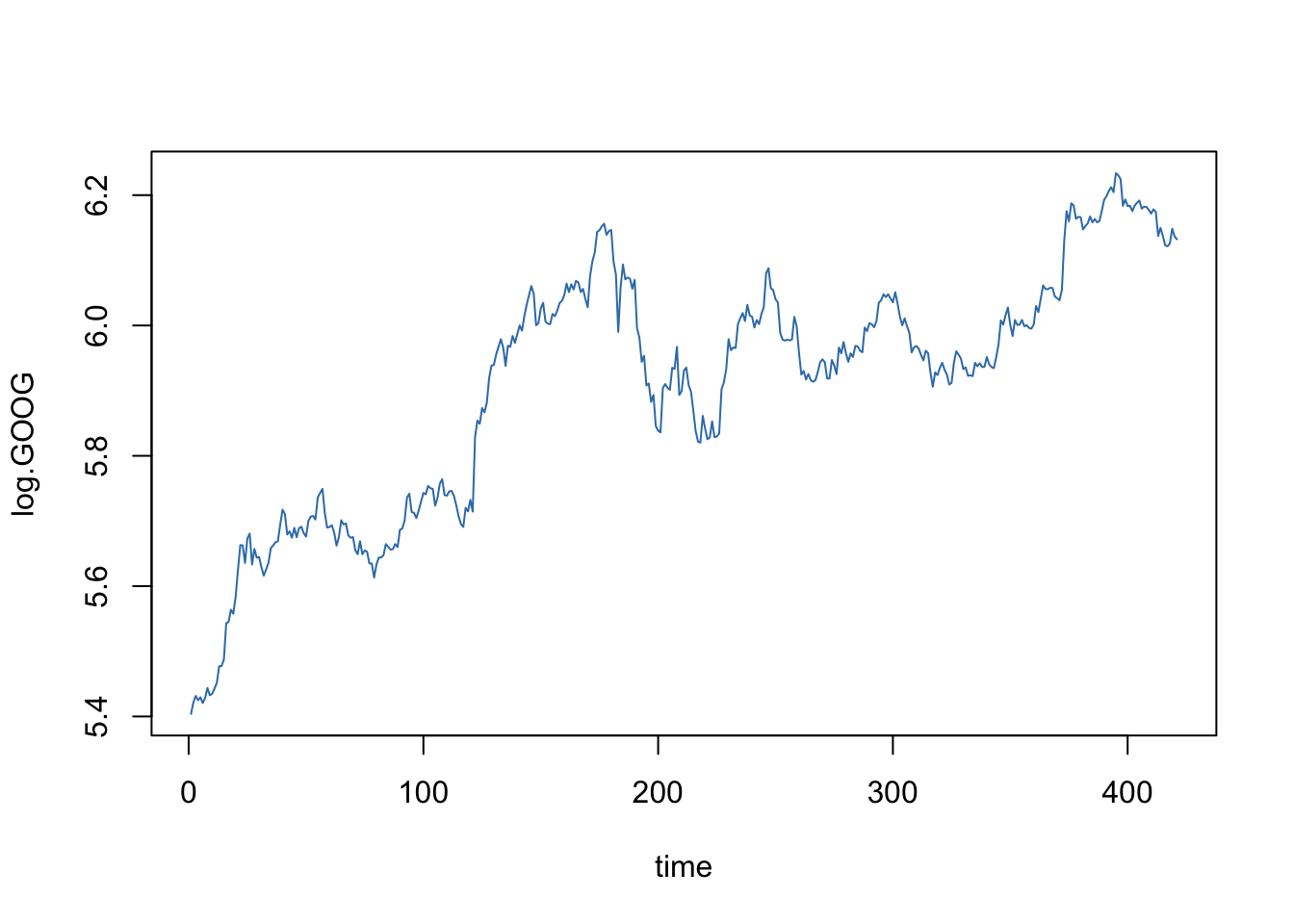``library("forecast")``
``Loading required package: zoo``
``````
Attaching package: 'zoo'``````
``````The following objects are masked from 'package:base':

as.Date, as.Date.numeric``````
``Loading required package: timeDate``
``Loading required package: methods``
``This is forecast 7.3 ``

Daily closing price: May 2, 2005 to December 29, 2006.

``````data <- read.csv("http://ptrckprry.com/course/forecasting/data/google.csv")
n <- length(GOOG)
time <- 1:n
plot(time, GOOG, type="l", col=2)``````## Log GOOG

``````log.GOOG <- log(GOOG)
plot(time, log.GOOG, type="l", col=2)````````Acf(log.GOOG)``